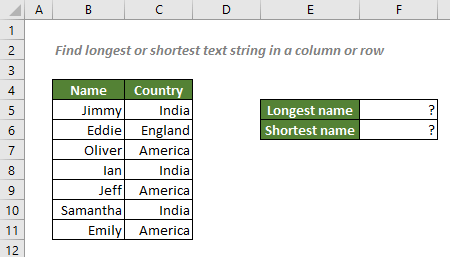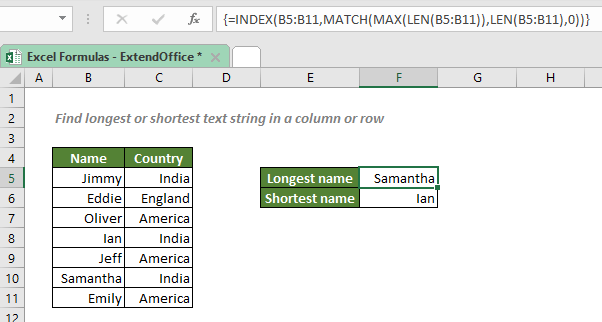## Find longest or shortest text string in a column or row

To look for the longest or shortest text string in a column or row in Excel, you can use theLEN and MAX or MIN functions together.#### How to find the longest or shortest string in a column or row in Excel?

To quickly find the longest or shortest name listed in the name range above, an INDEX, MATCH and LEN formula that incorporates the MAX or MIN function will help you in this way: The MAX or MIN function will retrieve the largest or smallest value in the range of string lengths provided by the LEN function. Then MATCH will locate the position of the largest or smallest value and INDEX will retrieve the value at the corresponding position.

#### Generic syntax

Find longest string: =INDEX(range,MATCH(MAX(LEN(range)),LEN(range),0))

Find shortest string: =INDEX(range,MATCH(MIN(LEN(range)),LEN(range),0))

√ Note: These are array formulas that require you to enter with Ctrl + Shift + Enter.

• range: The range where you want the combination formula to return the longest or shortest string from.

To find the longest or shortest name, please copy or enter the formulas below in the cell F5 or F6, and press Ctrl + Shift + Enter to get the results:

Find longest name (cell H5):
=INDEX(B5:B11,MATCH(MAX(LEN(B5:B11)),LEN(B5:B11),0))

Find shortest name (cell H6):
=INDEX(B5:B11,MATCH(MIN(LEN(B5:B11)),LEN(B5:B11),0))#### Explanation of the formula

Here we use the formula below as an example:

=INDEX(B5:B11,MATCH(MAX(LEN(B5:B11)),LEN(B5:B11),0))

• LEN(B5:B11): The LEN function returns an array of the lengths of each name in B5:B11 like this: {5;5;6;3;4;8;5}.
• MAX(LEN(B5:B11)) = MAX({5;5;6;3;4;8;5}): The MAX function then returns the largest value of the array {5;5;6;3;4;8;5}, which is 8.
• MATCH(MAX(LEN(B5:B11)),LEN(B5:B11),0) = MATCH(8,{5;5;6;3;4;8;5},0): The match_type 0 forces the MATCH function to find the position of the exact number 8 in the array {5;5;6;3;4;8;5}. So, it returns 6 since the number is at the 6th position.
• INDEX(B5:B11,MATCH(MAX(LEN(B5:B11)),LEN(B5:B11),0)) = INDEX(B5:B11,6): The INDEX function returns the 6th value in the name range B5:B11, which is Samantha.

#### Related functions

Excel INDEX function

The Excel INDEX function returns the displayed value based on a given position from a range or an array.

Excel MATCH function

The Excel MATCH function searches for a specific value in a range of cells, and returns the relative position of the value.

Excel LEN function

The LEN function returns the number of characters in a text string.

#### Related Formulas

Find longest text string with criteria

In this tutorial, we will talk about how to look for the longest text string in a column or row with criteria in Excel with the help of INDEX, MATCH, LEN and MAX functions.

Approximate match with INDEX and MATCH

There are times when we need to find approximate matches in Excel to evaluate employees' performance, grade students’ scores, calculate postage based on weight, etc. In this tutorial, we will talk about how to use the INDEX and MATCH functions to retrieve the results we need.

Lookup closest match value with multiple criteria

In some cases, you may need to lookup the closest or approximate match value based on more than one criteria. With the combination of INDEX, MATCH and IF functions, you can quickly get it done in Excel.

### The Best Office Productivity Tools

#### Kutools for Excel - Helps You To Stand Out From Crowd

 Popular Features: Find, Highlight or Identify Duplicates  |  Delete Blank Rows  |  Combine Columns or Cells without Losing Data  |  Round without Formula ... Super VLookup: Multiple Criteria  |  Multiple Value  |  Across Multi-Sheets  |  Fuzzy Lookup... Adv. Drop-down List: Easy Drop Down List  |  Dependent Drop Down List  |  Multi-select Drop Down List... Column Manager: Add a Specific Number of Columns  |  Move Columns  |  Toggle Visibility Status of Hidden Columns  |  Compare Columns to Select Same & Different Cells ... Featured Features: Grid Focus  |  Design View  |  Big Formula Bar  |  Workbook & Sheet Manager | Resource Library (Auto Text)  |  Date Picker  |  Combine Worksheets  |  Encrypt/Decrypt Cells  |  Send Emails by List  |  Super Filter  |  Special Filter (filter bold/italic/strikethrough...) ... Top 15 Toolsets:  12 Text Tools (Add Text, Remove Characters ...)  |  50+ Chart Types (Gantt Chart ...)  |  40+ Practical Formulas (Calculate age based on birthday ...)  |  19 Insertion Tools (Insert QR Code, Insert Picture from Path ...)  |  12 Conversion Tools (Numbers to Words, Currency Conversion ...)  |  7 Merge & Split Tools (Advanced Combine Rows, Split Excel Cells ...)  |  ... and more

Kutools for Excel Boasts Over 300 Features, Ensuring That What You Need is Just A Click Away...#### Office Tab - Enable Tabbed Reading and Editing in Microsoft Office (include Excel)

• One second to switch between dozens of open documents!
• Reduce hundreds of mouse clicks for you every day, say goodbye to mouse hand.
• Increases your productivity by 50% when viewing and editing multiple documents.
• Brings Efficient Tabs to Office (include Excel), Just Like Chrome, Edge and Firefox.